# Linearly-regular random process

A stationary stochastic process (in the wide sense)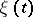,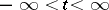, for which the regularity condition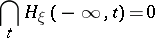is satisfied, where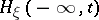is the mean square closed linear hull of the values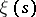,. (Here it is assumed that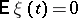.) Regularity implies the impossibility of a (linear) prediction of the processin the very distant future; more precisely, ifis the best linear prediction for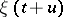with respect to the values,,thenA necessary and sufficient condition for regularity of a (one-dimensional) stationary process is the existence of a spectral density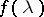such thatThe analytic conditions for regularity of multi-dimensional and infinite-dimensional stationary processes are more complicated. In the general case, when the spectral densityis a positive operator function in Hilbert space, the regularity condition is equivalent to the fact thatadmits a factorization of the form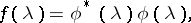where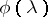,, is the boundary value of an operator function,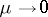, that is analytic in the lower half-plane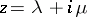,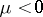.

Every processthat is stationary in the wide sense admits a decomposition into an orthogonal sum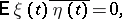whereis a linearly-regular process and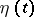is a linearly-singular process, that is, a stochastic process that is stationary in the wide sense and for which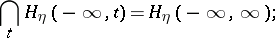also,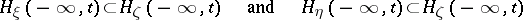for all.